## 2009 AIME I Math Jam

Go back to the Math Jam Archive

AoPS instructors discuss all 15 problems of the 2009 AIME I.

#### Facilitator: Dave Patrick

DPatrick18:59:22
Hello and welcome to the 2009 AIME I Math Jam!
DPatrick18:59:35
Before we get started I would like to take a moment to explain our virtual classroom to those who have not previously participated in a Math Jam or one of our online classes.
DPatrick18:59:53
The classroom is moderated, meaning that students can type into the classroom, but only the moderator (that's me) can choose a comment to drop into the classroom.
DPatrick19:00:09
This helps keep the class organized and on track. This also means that only well-written comments will be dropped into the classroom, so please take time writing responses that are complete and easy to read.
DPatrick19:00:32
There are a lot of students here! As I said, only well-written comments will be passed to the entire group. Please do not take it personally if your comments do not get posted, and please do not complain about it. This Math Jam to be much larger than our typical class, so please be patient---there are quite a few of you here tonight!
DPatrick19:00:50
Also, we won't be going through the math quite as thoroughly as we do in our classes -- I can't teach all the prerequisite material for every problem as we go (and certainly not to this many students -- this Math Jam won't be as orderly or as thorough as our typical online classes.)! If you do not know a particular concept necessary for a problem, I probably will not be able to explain it to you tonight.
DPatrick19:01:04
Please also remember that the purpose of this Math Jam is to work through the solutions to the AIME problems, and not to merely present the answers. "Working through the solutions" includes discussing problem-solving tactics.
DPatrick19:01:16
So please, when a problem is posted, do not simply respond with the answer. That's not why we're here. We're going to work through the problems step-by-step, and people who post comments that skip key steps or jump ahead in the problem, without providing explanation or motivation, are going to be ignored.
DPatrick19:01:27
I don't claim that the solutions that we'll present today are the "definitive" or "best" solutions to each problem. Many of the problems have more than one plausible approach. We don't have time to consider every possible approach to every problem.
DPatrick19:01:57
Also, these solutions are not necessarily the fastest, but these solutions are the ones that (to me) are the most logical or the ones that you'd be most likely to think of on your own.
DPatrick19:02:53
One last thing: if you're here for a regular AoPS Algebra class, this is the Math Jam, not your class. Please exit and reload the classroom when your class starts to get to the right place.
DPatrick19:03:00
Our agenda is to work through all 15 problems on the 2009 AIME I, in order.
DPatrick19:03:09
So let's get started with #1:
DPatrick19:03:14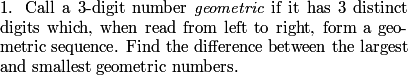DPatrick19:04:15
As you can see, I will pop each problem up to the top of the screen, so it will be constantly displayed while we work through it.
DPatrick19:04:31
You can click and drag the horizontal gray bar near the top to make that window smaller or larger.
1=219:04:56
The least has a hundreds digit of 1 and the greatest has a hundreds digit of nine, so we need to find the least geometric number with a hundreds digit of 1 and the greatest with a hundreds digit of 9.
DPatrick19:05:10
Right. We want to try for a largest number starting with 9 and a smallest number starting with 1.
DPatrick19:05:28
The smallest number is easier.
DPatrick19:05:52
If the first digit is 1, and the middle digit is r, what is the units digits?
rubik19:06:02
r^2
AIME1519:06:02
r^2
DPatrick19:06:09
If the middle digit is r then the last digit has to be r^2.
chessmaster719:06:27
smallest possible value would be 124 then
pinkmuskrat19:06:27
r must be smalles; is 2
DPatrick19:06:42
To make this as small as possible, we choose r=2, so the smallest geometric number is 124.
DPatrick19:06:58
How about the largest? If the hundreds digit is 9, and the middle digit is d, what is the smallest digit?
ytrewq19:07:22
d^2/9
bpgbcg19:07:22
d^2/9
DPatrick19:07:44
The ratio between the first two digits is d/9, so the smallest digit is d * (d/9) = d^2/9.
DPatrick19:08:10
What's the largest d (other than 9) such that d^2/9 is an integer?
ytrewq19:08:46
d must be divisible by 3, so d=6 is the largest
tinytim19:08:46
d must be a multiple of 3.
DPatrick19:08:52
d must be a multiple of 3, so we want d=6.
DPatrick19:09:03
Thus 964 is the largest geometric integer.
e^i_penguin19:09:13
DPatrick19:09:23
Thus, the answer is 964 - 124 = 840.
DPatrick19:09:57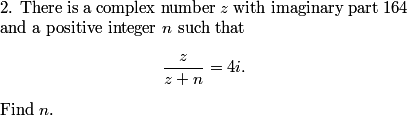AIME1519:10:26
let z=a+164i
e^i_penguin19:10:26
express z as a+164i where a is a real number
blackbelt1425319:10:26
let z=a+164i
DPatrick19:10:35
We are given that z = a + 164i for some a.
DPatrick19:10:45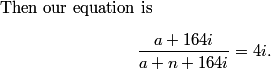panjia12319:11:17
get rid of the fraction
ytrewq19:11:17
multiply by a+n+164i
VDLmath19:11:17
simplify by getting rid of the denominator
Nerd_of_the_Ages19:11:17
Multiply out the denominator.
DPatrick19:11:33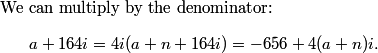mile2319:11:53
get a = -656
1=219:11:53
a=-656
DPatrick19:12:06
Comparing the real parts of this, we see that a = -656.
happysunnyshine19:12:18
substitute a =-656 into the equation
DPatrick19:12:41
We can plug that in, and then equate the imaginary parts:
DPatrick19:12:48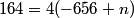alligator11219:13:16
41=-656+n, n=697
drizzt12319:13:16
so n=697
yasuaka200819:13:16
n = 41 + 656 = 697
Ttocs4519:13:16
Since -656+n=41, n=697
DPatrick19:13:21
Divide by 4 to get 41 = -656 + n.
DPatrick19:13:29
Thus n = 656 + 41 = 697.
DPatrick19:13:54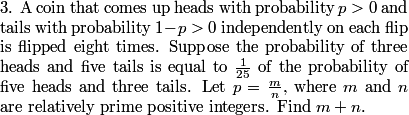Octahedr0n19:14:27
Set up an equation relating the probabilities.
rubik19:14:27
write an equation:
jeffreyyan819:14:27
set up an equation
harbinger19:14:27
Find the probabilities with respect to p
DPatrick19:14:43
We can just calculate these two probabilities directly, and set up an equation for p.
DPatrick19:14:53
What's the probability of 3 heads and 5 tails?
tinytim19:15:23
(8C3)p^3(1-p)^5
AIME1519:15:23
8C3 * p^3 * (1-p)^5
rubik19:15:23
8C3 * p^3 * (p-1)^5
mathking12319:15:23
8C3*p^3*(1-p)^5
DPatrick19:15:31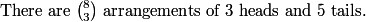DPatrick19:15:44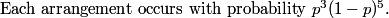DPatrick19:15:57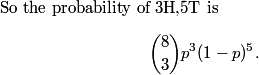DPatrick19:16:11
What's the probability of 5 heads and 3 tails?
Welovemath19:16:43
8C3 * p^5 * (1-p)^3
vallon2219:16:43
8C3 * p^5 * (1-p)^3
moplam19:16:43
(8C5)p^5(1-p)^3
DPatrick19:17:03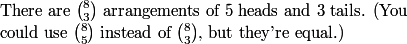DPatrick19:17:15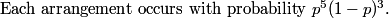DPatrick19:17:24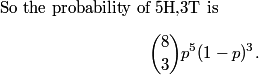VDLmath19:17:58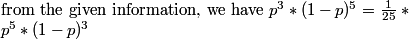rubik19:17:58
so the equation is 8C3 * p^3 * (1-p)^5 = 1/25 * p^5 * (1-p)^3
vallon2219:17:58
we are told the probability of 3 heads and 5 tails is 1/25 the probability of 5 heads and 3 tails, so we can set up an equation
DPatrick19:18:14
Right. We're told the former (3H,5T) is 1/25 of the latter (5H,3T).
DPatrick19:18:20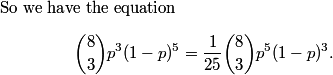tinytim19:18:37
we can divide both sides by (8C3)p^3(1-p)^3
DanielC19:18:38
divide by 8C3 *p^3* (1-p)^3
Kevin Zhang19:18:38
divide out (8C3)*p^3*(1-p)^3 from both sides of the resulting equation
DPatrick19:18:42
A lot of stuff cancels!
DPatrick19:18:49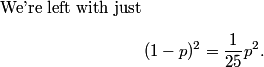stevenmeow19:19:11
square root
ytrewq19:19:11
take the square root
blackbelt1425319:19:11
square root both sides (since both p and 1-p are positive)
DPatrick19:19:15
Everything is positive, so we can take square roots:
DPatrick19:19:25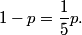BOGTRO19:19:41
and p=5/6
DPatrick19:19:46
This solves to give p = 5/6, so the answer is 5+6 = 011.
DPatrick19:20:07
At this point, I would do a "sanity check" to make sure the answer is reasonable.
piisdelicious19:20:32
it is smaller than 1
tinytim19:20:40
It is because from the given we can see that heads is more likely than tails,
DPatrick19:20:42
5H3T is 25 times more likely than 3H5T. So the probability of heads should be much larger than 1/2, but of course less than 1. Thus 5/6 makes sense.
MathForFun19:21:02
why wouldn't it be just 11, instead of 011?
DPatrick19:21:04
DPatrick19:21:30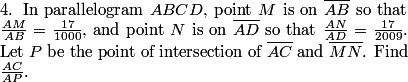e^i_penguin19:22:05
Assume the parallelogram is a rectangle WLOG
DanielC19:22:06
put it on a rectangle
rubik19:22:06
assume it's a rectangle?
FibonacciFan19:22:06
the problem implies that ANY parallelogram with those ratios will give the same answer, so just make it a rectangle
DPatrick19:22:15
True: we have a bit of freedom, so we might as well make life easy!
DPatrick19:22:25
I found it easiest to assume that ABCD is a rectangle, with AB = 1000 and AD = 2009.
DPatrick19:22:46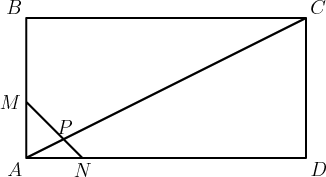DPatrick19:23:18
(If you didn't think of this, I'll show you in a moment how you can do it with a non-rectangular parallelogram.)
e^i_penguin19:23:46
Solve a coordinate system
stevenmeow19:23:46
MN has equation y=17-x, and AC has equation 1000x/2009
bpgbcg19:23:46
Then MN has equation x+y=17, and AC has equation 1000/2009*x=y.
DPatrick19:23:57
We can set it up easily with coordinates with A = (0,0), B = (0,1000), C = (2009,1000), and D = (2009,0).
DPatrick19:24:08
Then M = (0,17) and N = (17,0).
DPatrick19:24:17
(Of course, the image is not to scale!)
DPatrick19:24:52
We then see that point P is the intersection of the lines x+y = 17 and y = (1000/2009)x.
DPatrick19:25:25
What's easiest from here?
drizzt12319:25:50
substitute y as 1000/2009 x into x+y=17
stevenmeow19:25:50
substitution
FantasyLover19:25:50
substitute
DPatrick19:26:05
We can just plug the second equation into the first, and solve for x.
DPatrick19:26:17
x + (1000/2009)x = 17
DPatrick19:26:27
(3009/2009)x = 17
DPatrick19:26:34
x = 17*(2009/3009).
DPatrick19:26:38
How do we finish from here?
e^i_penguin19:27:12
BenM19:27:12
DPatrick19:27:25
We can just compute the ratio of the x-coordinate of C to the x-coordinate of P. (In particular, we don't need to solve for y.)
DPatrick19:27:42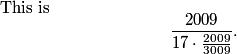e^i_penguin19:28:06
so, we have the ratio as 3009/17 = 177
brightzhu19:28:06
After cancelling its 3009/17=177
DPatrick19:28:13
This equals 3009/17 = 177.
DPatrick19:28:48
Quick announcement before we continue:
DPatrick19:29:06
If you're here for a regular AoPS Algebra class, you're in the wrong room. Please logout of the classroom and reconnect to get to your class.
tinytim19:29:12
Couldn't we also do it without coordinates using similar parallelograms?
DPatrick19:29:21
Correct. The problem states that it works for all parallelograms, so let's quickly see the proof of that.
DPatrick19:29:30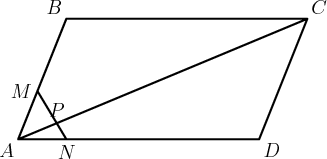DPatrick19:30:11
To use the ratios, we need similar triangles.
drizzt12319:30:35
draw a line parallel to BA from N to BC
DPatrick19:30:50
That works; I did essentially the same thing.
DPatrick19:31:15
We want to be able to use the ratios AM/AB and AN/AD. In particular, to get that first ratio, we want AM and AB to be corresponding sides of similar triangles.
DPatrick19:31:21
So we draw MQ parallel to BC:
DPatrick19:31:27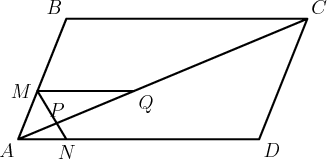DPatrick19:31:41
Now AMQ and ABC are similar.
DPatrick19:32:12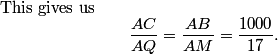DPatrick19:32:18
Now what?
DPatrick19:32:42
What other triangles are similar?
r15s11z55y89w2119:33:01
MQP and NAP
vallon2219:33:01
PMQ and PNA
DPatrick19:33:06
PMQ and PNA are also similar.
drizzt12319:33:55
so AP/PQ=AN/MQ
happysunnyshine19:34:02
pq/ap=Mq/AN
DPatrick19:34:21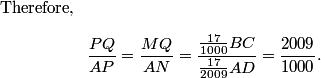moplam19:34:55
We can do PQ/AP=(AQ-AP)/AP=AQ/AP-1
DPatrick19:35:14
Right, we really want AC/AP, so we need AQ/AP (to multiply by AC/AQ that we computed previously).
DPatrick19:35:23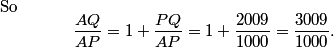bpgbcg19:36:27
AC/AP=AC/AQ*AQ/AP=3009/1000*1000/17=177.
Yongyi78119:36:27
So we have 3009/1000 * 1000/17 = 3009/17 = 177
moplam19:36:30
AC/AP=(AQ/AP)*(AC/AQ)=3009/1000*1000/17=3009/17=177
DPatrick19:36:33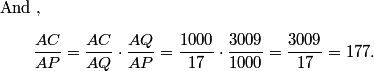DPatrick19:36:48
Hence the answer is 177, and it works for all parallelograms.
stevenmeow19:37:02
I think we could have assumed the parallelogram is a line with AC=3009, MN=0, and AP=17.
Kevin Zhang19:37:02
There's a really easy way to solve this problem! Set A and C are 0 degrees; still technically a parallelogram! After that, you can see that AB + BC=3009. AP=17, so 3009/17=177
DPatrick19:37:24
OK, if you're confident doing this it does happen to work, but this is a much more dangerous assumption.
DPatrick19:37:37
There are other solutions methods too, but let's move on.
DPatrick19:37:51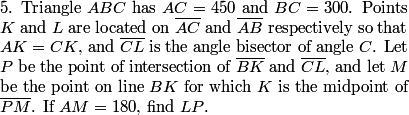moplam19:38:12
Well, we should draw it first
stevenmeow19:38:15
We should first draw a diagram!
DPatrick19:38:23
Half the battle here is drawing an accurate diagram using the given information.
DPatrick19:38:30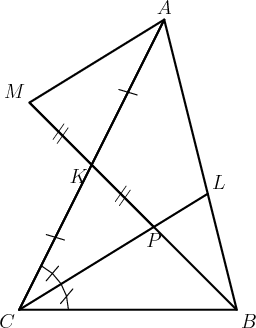tinytim19:39:27
By SAS Congruency, MKA is congruent to PKC
AMK and KCP are congruent
DPatrick19:39:35
The key thing to notice is that triangles AKM and CKP are congruent.
bowser19:39:56
triangle AMK and CPK are congruent so MA and CL are parallel
DiscreetFourierTransform19:40:02
Which generates similar triangles ABM and LBP
DPatrick19:40:12
Exactly. This means that AM and CP (and hence CL) are parallel.
DPatrick19:40:23
This means BPL and BMA are similar.
DPatrick19:40:36
So if we can find the ratio PL/MA, we'd be done, since we know MA = 180.
DPatrick19:40:43
Fortunately, that ratio is in the diagram, and there's one piece of data we haven't used yet.
Yongyi78119:40:58
Angle bisector theorem
policecap19:40:59
well you can use angle bisector thm
BenM19:40:59
Angle bisector
DPatrick19:41:11
Right. We haven't used the fact that CL is an angle bisector.
DPatrick19:41:17
This lets us use the Angle Bisector Theorem!
moplam19:41:35
AL/LB=CA/CB
DPatrick19:41:49
Right. That's the theorem (as applied to our problem).
DPatrick19:42:01
This theorem tells us BL/AL = BC/AC = 300/450 = 2/3.
DPatrick19:42:18
But what we need is BL/AB.
Fermat160119:42:41
Because sof the similar triangles, PL/MA = 2/5
panjia12319:42:41
That's 2/(2+3)=2/5
tinytim19:42:41
Its just 2/(3+2)
DPatrick19:42:45
Right.
DPatrick19:43:07
The other way to think of it is that the ratio BL/LA = 2/3. So BL is 2/5 of the total of AB (and AL is the other 3/5).
Fermat160119:43:30
BL/AB = 2/5 = PL/MA = x/180
tinytim19:43:30
so LP=2/5MA=2/5*180=72
DPatrick19:43:49
Exactly: to finish, we have PL/MA = BL/BA = 2/5.
DPatrick19:43:55
So PL = 2/5(AM) = 2/5(180) = 072.
DPatrick19:44:27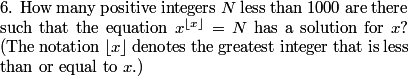stevenmeow19:45:01
We should do casework on the floor of x.
DiscreetFourierTransform19:45:01
Obviously you're going to be taking things to an integer power
DPatrick19:45:13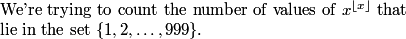DPatrick19:45:36
We can categorize the values based on the integer [x].
DPatrick19:45:41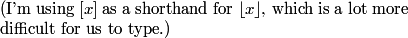sup3rcrash3r19:45:57
examine each case where the floor is 1-4
r15s11z55y89w2119:45:57
floor of x can be 1,2,3,or 4
DPatrick19:46:14
Well, it would be nice to rule out [x] < 1 first before jumping ahead.
Kevin Zhang19:46:31
x^0 is 1
mile2319:46:35
if [x] = 0, the N= 1
DPatrick19:46:52
If [x] = 0, then the function is 1 no matter what.
vallon2219:47:15
we should prove that x cannot be negative. if x < -1, then the result would be a fraction. if -1 < x < 0, then the result would be x^-1, which would be negative. since N is a positive integer, x cannot be that.
DPatrick19:47:32
Right. If -1 <= x < 0, then the function is negative, and if x < -1, then the value of the function is between -1 and 1, so we can ignore all those.
DPatrick19:47:50
So now we can look at the cases where [x] is a positive integer.
tinytim19:48:04
if [x]=1, then N also equals 1
mile2319:48:04
for [x] = 1, the only x that works is x =1
DPatrick19:48:28
If [x] = 1, then 1 <= x < 2. The function runs from 1^1 up to (but not equal to) 2^1. So it only hits the positive integer 1.
DPatrick19:48:38
DPatrick19:48:42
What happens if [x] = 2?
policecap19:49:07
if [x]=2, then N=4,5,6,7,8
Bobby Jacobs19:49:08
If [x]=2, then 4, 5, 6, 7, and 8 work.
panjia12319:49:08
2^2 up to but not equal to 3^2
blackbelt1425319:49:08
then it hits 4-8
DPatrick19:49:12
We have 2 <= x < 3.
DPatrick19:49:20
So the function runs from 2^2 up to (but not equal to) 3^2.
DPatrick19:49:32
Hence it hits all integers 4 <= N < 9.
DPatrick19:49:45
That's 9-4 = 5 values of N.
DPatrick19:49:55
What happens if [x] = 3?
Bobby Jacobs19:50:31
For [x]=3, 27<=N<64
ytrewq19:50:31
if [x]=3 then 3^3<=N<4^3
moplam19:50:31
For [x]=3, we have everything from 3^3 to 4^3 exclusing 4^3
DPatrick19:50:37
Right. We have 3 <= x < 4.
DPatrick19:50:50
So 3^3 <= N < 4^3. In other words, 27 <= N < 64.
DPatrick19:51:03
That's 64-27 = 37 values of N.
DPatrick19:51:12
(To summarize, we have 1 + 5 + 37 values of N so far.)
DPatrick19:51:21
What happens if [x] = 4?
alligator11219:51:42
for [x]=4, we have 4^4<=N<5^4
mathking12319:51:42
the function runs from 4^4 up to (but not equal to) 5^4
Spring19:51:42
4^4<=N<5^4
Caelestor19:51:42
If [x]=4, then 4^4<=N<5^4
DPatrick19:51:49
We have 4 <= x < 5. So 4^4 <= N < 5^4.
DPatrick19:51:59
This gives 256 <= N < 625.
bpgbcg19:52:10
625-256=369 possibilities
UberYoda19:52:10
this gives 369 values of N
DPatrick19:52:14
So that's 625 - 256 = 369 more values of N.
DPatrick19:52:21
(That's 1+5+37+369 values so far.)
sup3rcrash3r19:52:35
[x] = 5 does not work because 5^5 is larger than 999
tinytim19:52:35
We can stop there because [x]=5 gives values greater than 999
alligator11219:52:35
if [x]=5, there is none because 5^5 is in the 3 thousands and is not less than 1000
DPatrick19:52:52
Right. 5^5 = 3125 is way too big. So any [x] >= 5 will give a number that's too big. Thus we've got them all.
e^i_penguin19:52:59
DPatrick19:53:04
Thus, we have 1 + 5 + 37 + 369 = 412 total values of N.
DPatrick19:53:59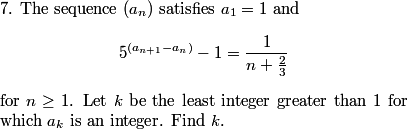e^i_penguin19:54:31
Try the first few a_n to find a pattern or get a feel for the sequence
tinytim19:54:31
first find a_2 to try and start a pattern.
1=219:54:31
Try some values of a_n where n is greater than 1 yet small.
Yoshi19:54:31
We neeed to find a pattern
DPatrick19:54:55
We could try computing the values of the sequence, but I'd shy away from that, as we suspect that's bound to be ugly: the problem implies a lot of them are not integers.
dnkywin19:55:12
isolate the exponent
DPatrick19:55:21
Yes, we don't like the recursive part of our equation appearing in the exponent.
bpgbcg19:55:29
moplam19:55:29
let move 1 to the RHS
Caelestor19:55:29
Move the 1 to the right side
DPatrick19:55:35
We can move the 1 to the other side first:
DPatrick19:55:40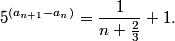ytrewq19:56:00
simplfy the fraction
DiscreetFourierTransform19:56:01
and re-write it as (n+5/3)/(n+2/3)
blackbelt1425319:56:03
simplify the fraction...that doesn't look nice on the RHS.
DPatrick19:56:19
You might jump straight to taking the logarithm here, but I'd clean up the right side first.
DPatrick19:56:24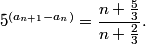Caelestor19:56:31
(3n+5)/(3n+2) I think
yasuaka200819:56:32
RHS simplifies to (3n + 5)/(3n+2)
zappy0719:56:32
or (3n+5)/(3n+2)
DPatrick19:56:40
Yes, it's still icky. Let's make it nicer:
DPatrick19:56:45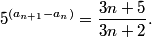tinytim19:57:02
now we take the base 5 log
DiscreetFourierTransform19:57:02
Take logs now.
Caelestor19:57:02
DPatrick19:57:08
Now we can take logs of both sides:
DPatrick19:57:14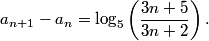DiscreetFourierTransform19:57:26
Use the difference of logs formula
moplam19:57:26
seperate the log
DPatrick19:57:31
We can break up the expression on the right side:
DPatrick19:57:38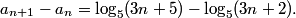DPatrick19:58:26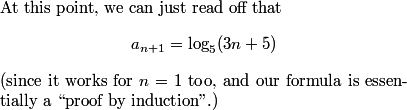DPatrick19:58:44
(I should say it works for n=0)
DanielC19:58:53
now telescope it
DPatrick19:59:06
Or we could prove this by summing them all up, and noticing that it telescopes.
DPatrick19:59:34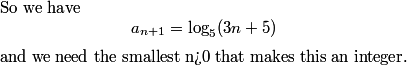DPatrick19:59:42
oops, that should be n>0.
blackbelt1425319:59:54
now we need 3n+5 to be a integer power of 5.
BenM19:59:54
now set 3n+5 equal to powers of 5
mathquests19:59:54
so find the n for which 3n+5 is a power of 5
DPatrick20:00:00
We need 3n+5 to be a power of 5.
FantasyLover20:00:26
3n+5=25 doesn't work and try 125 which works
Ttocs4520:00:27
125 is 5^3
TheWorstPlayer20:00:27
It should be the smallest, which is 125.
DPatrick20:00:46
We can't make it equal 5 (since n>0) or 25 (since then n is not an integer), but we can make it equal 125 by setting n=40.
Kevin Zhang20:01:02
moplam20:01:02
3n+5=125-->n=40, an+1=a41
DPatrick20:01:13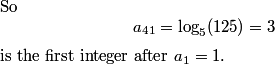DPatrick20:01:44
DPatrick20:02:20
Next comes a run of 4 counting problems in a row.
DPatrick20:02:31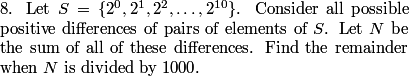DPatrick20:02:59
What's the key idea here?
bpgbcg20:03:16
See how many times each element is added or subtracted.
sup3rcrash3r20:03:16
find a pattern
DPatrick20:03:52
There are a number of ways to do this (including brute-force listing all 55 pairs!), but I found it easy to: instead of trying to determine all the "pairs", we instead just keep track of how many times each element gets added and subtracted.
DPatrick20:04:03
For example, how many times does 2^10 get added/subtracted?
blackbelt1425320:04:31
BenM20:04:31
Welovemath20:04:31
sup3rcrash3r20:04:31
it gets added 10 times and subtracted 0 times
DPatrick20:04:42
It's the larger element of 10 pairs, so it gets added 10 times. It's the smaller element of 0 pairs, so it gets subtracted 0 times.
DPatrick20:04:56
How many times does 2^9 get added/subtracted?
BenM20:05:13
blackbelt1425320:05:13
DPatrick20:05:20
It's the larger element of 9 pairs, so it gets added 9 times. It's the smaller element of 1 pair, so it gets subtracted 1 time. Thus it gets added a net 8 times.
DPatrick20:05:27
How does this pattern continue?
and it continues going down by 2s
Welovemath20:05:56
6,4,2,0,-2,-4,-6,-8,-10
fortenforge20:05:56
10 8 6 4 2 so on
DPatrick20:06:03
2^8 gets added a net 8-2 = 6 times. 2^7 gets added a net 7-3 = 4 times. 2^6 gets added a net 6-4 = 2 times. 2^5 gets added a net 5-5 = 0 times. 2^4 gets added a net 4-6 = -2 times, so it gets subtracted 2 times. and so on... up to 2^0 gets subtracted 10 times.
DPatrick20:06:25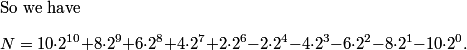tinytim20:07:04
now its just painful computation...
Fermat160120:07:05
brute force?
poseidon20:07:05
now we solve
DPatrick20:07:10
These numbers are relatively easy to crunch out by hand, especially when we keep in mind that we only care about them mod 1000.
DPatrick20:07:25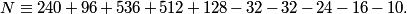DPatrick20:07:47
You can also save a little time in this calculation by noting, for example, that 536 + 512 - 32 - 16 = 0 (mod 1000), so that cancels 4 of the terms (including the two largest terms).
AIME_is_hard20:08:04
N=398 mod 1000
DPatrick20:08:16
It works out to 398 as our answer.
DPatrick20:08:45
There are certainly slicker algebraic tricks you can us on this sum, or you can even set it up as a recursion.
DPatrick20:09:18
I need a slight break from typing, so let's take a 5-minute break now. With 150+ students I need to leave the chat off during the break. We'll resume at around :15 past the hour.
DPatrick20:14:17
OK, we're back! :)
DPatrick20:14:32
As we get into problems 9-15, the math will occassionally get more technical.
DPatrick20:14:50
As I said at the beginning, I can't teach all the prerequisite material for every problem as we go (and certainly not to this many students -- this Math Jam won't be as orderly or as thorough as our typical online classes.)! If you do not know a particular concept necessary for a problem, I probably will not be able to explain it to you tonight.
DPatrick20:15:13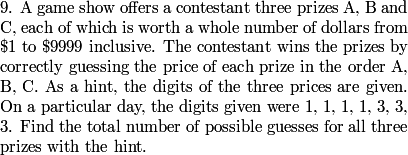stevenmeow20:16:27
Each permutation of prizez corresponds to a permutation of the digits 1,1,1,1,3,3,3 with 2 commas inserted in one of 12 ways
Fermat160120:16:27
we can make 7 spots for the numbers and split them into the three prize amounts.
DiscreetFourierTransform20:16:27
First perhaps, attempt a balls and urns-type strategy.
panjia12320:16:27
first find the number of ways the numbers can be arranged
mathmagus20:16:27
Find the possible permutations of the numbers, then find the groupings.
DPatrick20:16:35
Right...I'll elaborate.
DPatrick20:16:52
To use these digits to construct a guess, we have a two-step process.
DPatrick20:17:02
First, we arrange the digits left-to-right. Second, we separate the digits into group A, group B, and group C.
DPatrick20:17:21
For example, 31 | 133 | 11 would be a possible guess, where we first order the digits as 3113311, and then insert two dividers. The guess is 31 for A, 133 for B, and 11 for C.
DPatrick20:17:35
How many ways to arrange the digits?
AIME20:18:00
First, we know that there are 7 choose 3= 35 different arrangements of four ones and three threes.
stevenmeow20:18:00
There are 35 orderings of the digits (7C3)
tinytim20:18:00
to arrange the digits we have 7C3=25 ways
mathquests20:18:00
there are 7 choose 3 = 35 ways to arrange the digits
DPatrick20:18:20
It's C(7,3) = 35. (We have 7 digits, and we must choose 3 of them to be threes.)
DPatrick20:18:27
How many ways to separate them into 3 groups?
mathquests20:19:24
there are 15 ways to separate them
stevenmeow20:19:24
There are 15 ways to insert 2 commas (6C2) but 3 of these ways produce a 5-digit prize. The answer is 15-3=12
brightzhu20:19:24
from balls and urns 15 minus 3 for (5,1,1)(1,5,1)(1,1,5)
DPatrick20:19:26
Right.
DPatrick20:19:42
To seperate them into 3 nonempty groups, we have to insert 2 "dividers" into the 6 "slots" between the digits.
DPatrick20:19:57
So that's C(6,2) = 15 choices for our dividers.
DPatrick20:20:08
But 3 of these choices illegally produce a 5-digit guess.
DPatrick20:20:23
(For example, 3 | 11331 | 1 would be illegal in our original example.)
DPatrick20:20:35
So we really only have 12 legal choices of dividers.
e^i_penguin20:20:51
Final Answer = 35 * 12 = 420
blackbelt1425320:20:51
so the answer is 12*35=420, since each arrangement of the digits and dividers leads to a distinct guess.
DiscreetFourierTransform20:20:51
Which is just 12*35 = 420 ways to do it.
Fermat160120:20:51
So we multiply 7C3 * (6C2-3) = 35*12 = 420.
DPatrick20:20:58
Therefore, the number of guesses is 35 * 12 = 420.
DPatrick20:21:56
The second part of this -- inserting "dividers" to seperate a row of items into groups -- is a very common technique called a "distribution". (This is also sometimes called "balls and urns".)
DPatrick20:22:14
You can look it up later if you're not familiar with it. It almost always comes up in at least one AIME problem.
DPatrick20:22:27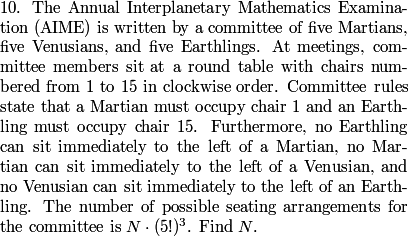DPatrick20:22:43
(It took me forever to type that up on Tuesday.)
DPatrick20:23:00
What's the significance of the (5!)^3 term in the total?
tenniskidperson320:23:24
It accounts for the permutations in the species.
mathmagus20:23:24
The number of arrangements of each race.
algebra200020:23:24
the ways to arrange the 5 members of each race
benzi45520:23:24
it accounts for the distinguishability of the members of each type
blackbelt1425320:23:24
each group of earthlings, etc. have 5! arrangements internally
DPatrick20:23:32
Once we assign seats to each planet (that is, decide which 5 seats are Martian, which 5 are Venusian, and which 5 are Earthling), we have 5! ways to assign each planet's 5 people to their seats.
DPatrick20:23:48
So N just counts the number of ways to assign the seats to planets, and we don't have to worry about the specific individuals.
DiscreetFourierTransform20:24:09
The restriction that there is a martian in chair one and an earthling in chair fifteen essentially reduces this problem to dealing with a straight line.
blackbelt1425320:24:28
this problem is basically asking for the # of ways to arrange them in a line, since the chairs are numbered, right?
DPatrick20:24:49
Correct. Fixing chairs #1 and #15 removes any sort of rotational symmetry. We just have to worry about the order, starting at 1 and going to 16.
DPatrick20:24:50
..15.
DPatrick20:25:07
Now what?
rubik20:25:37
the pattern is MEV
mathmagus20:25:37
We know that there can't be arrangements of EM, MV, or VE.
Bobby Jacobs20:25:37
No Earthling can be to the left of a Martian...
DPatrick20:25:48
Going around the table, starting at 1 and ending at 15, the planets have to be seated (Mars)(Venus)(Earth)(Mars)(Venus)(Earth)...
DPatrick20:25:59
In other words, there's a group of 1 or more Martians, followed by a group of 1 or more Venusians, followed by a group of 1 or more Earthlings, repeated as necessary.
stevenmeow20:26:21
We should do casework on the number of blocks of martians
meenamathgirl20:26:21
casework based on how many of these appear
DPatrick20:26:45
Right. We're going to have some number of MVE blocks. We have to (a) decide how many blocks, then (b) allocate the number of seats in each block.
Bobby Jacobs20:27:06
Actually, it's (Mars)(Earth)(Venus)(Mars)(Earth)(Venus)...
DPatrick20:27:15
I think you're right. I have it backward.
bpgbcg20:27:48
I thought you had it right the first time.
DPatrick20:28:00
Actually, I think I did too.
BOGTRO20:28:08
I don't think it matters
dnkywin20:28:08
it doesn't matter
DPatrick20:28:23
It definitely does matter, since we start with M (#1) and end with E (#15).
DPatrick20:28:59
I had it right the first time: the non-Mars person next in line must be Venus (not Earth), and so on.
DPatrick20:29:16
It's a bit confusing because when we write it as a line, "left" becomes "right".
DPatrick20:29:36
So the pattern reading clockwise is MVEMVE... ending at E.
meenamathgirl20:29:47
because you face inward to a round table
DPatrick20:29:58
Right, the people are facing towards the center.
DPatrick20:30:11
Anyway, I think we all agree now that the pattern is a number of blocks of MVE.
DPatrick20:30:21
And now we can do the casework.
DPatrick20:30:32
If there's 1 block, how many ways to allocate seats?
Fermat160120:30:55
Right... so if there is only one block, there is only one combination (5-5-5)
moplam20:30:55
1
Bobby Jacobs20:30:55
1
sup3rcrash3r20:30:55
one
DPatrick20:31:00
Only 1. We must have 5Ms, then 5Vs, then 5Es.
DPatrick20:31:08
Fermat160120:31:29
For 2 blocks, we can have 14, 23, 32, or 41 for each race so 64
blackbelt1425320:31:29
4*4*4=64
tinytim20:31:29
4^3
benzi45520:31:29
4*4*4 = 64
DPatrick20:31:45
For each planet, we must choose between 1 and 4 to be in the first group, and the rest go to the second group.
DPatrick20:31:54
So 4 choices for each planet, hence 4^3 = 64 choices overall.
DPatrick20:32:03
DPatrick20:32:30
algebra200020:33:22
there would be 4 places to place 2 "dividers" for each race which is 4 choose 2=6, so 6*6*6=216
blackbelt1425320:33:22
on in each of the 3 groups, then the remaining 2 can go into the groups in 6 ways, so 6*6*6=216
tinytim20:33:22
we must distribute 5 to 3 groups where each group must have at least 1 so the number of ways to do this, by balls and urns, is (4C2)
DPatrick20:33:34
Right, you can think of this again as a "balls and urns" distribution problem.
DPatrick20:34:04
We can distribute 5 people into 3 nonempty groups by inserting 2 "dividers" in the 4 "slots" between them, so there are C(4,2) = 6 ways (for each species).
DPatrick20:34:26
If this seems unnecessarily complication, then we can reason that for each planet, we have two possibilities: 2,2,1 or 1,1,3.
DPatrick20:34:33
In each possibility, we must choose which of the 3 groups is the "odd group out" (the 1 of 2,2,1 or the 3 or 1,1,3).
DPatrick20:34:40
So there are 6 choices for each planet.
sup3rcrash3r20:34:48
there are 3 races, so 6^3
DPatrick20:35:00
Right, hence 6^3 = 256 choices overall in this case.
DPatrick20:35:07
DPatrick20:35:21
(oops, 6^3 = 216)
Fermat160120:35:48
For 4 blocks, we must have 1-1-1-2 (some order), and there are 4 places to put the 2. so 64 again
CA Math20:35:48
We choose one block to have 2 people, the rest have one.
blackbelt1425320:35:48
4*4*4=64. one go in each group, then the remaining one goes into 1 of 4 groups
DPatrick20:36:02
For each species, one group gets 2, the rest get 1. So 4 choices for which group gets 2 people.
DPatrick20:36:09
So 4 choices for each planet, hence 4^3 = 64 choices overall.
DPatrick20:36:24
tinytim20:36:38
for 5, it must go MVEMVEMVEMVEMVE, so only 1 way.
Fermat160120:36:38
1-1-1-1-1 (1 combo)
moplam20:36:38
There's only 1 way
happysunnyshine20:36:38
1-1-1-1-1, only i
DPatrick20:36:43
No choices here: it must be MVEMVEMVEMVEMVE. So only 1 possibility.
kstan01320:37:14
1 + 64 + 216 + 64 + 1 = 346
CA Math20:37:14
1^3+4^3+6^3+4^3+1^3=346 ways
DPatrick20:37:16
So we sum the cases to get our answer: 1 + 64 + 216 + 64 + 1 = 346.
ytrewq20:37:33
It's the cubes of the 4th row of Pascal's Triangle
DPatrick20:37:53
It is indeed, and you can see if you can use this observation to generalize the problem.
DPatrick20:38:09
Let's move on to the final third of the contest...
DPatrick20:38:15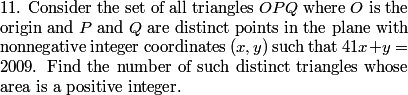DPatrick20:39:25
Before we get into it: if you know the Shoelace Theorem, you might use it. But it is totally unnecessary, as we will see. (And if you don't know it, you can look it up; I'm not going to explain it here.)
tinytim20:39:32
graph it first...
projectstartrek20:39:32
set up a coordinate system
all4math20:39:32
/////////////////////draw a coordinate plane
DPatrick20:39:40
A quick sketch might be a good way to start:
DPatrick20:39:47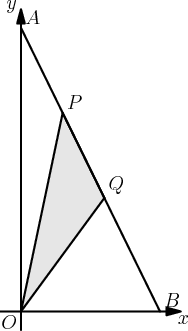DPatrick20:39:59
(Picture not to scale of course!)
gh62520:40:15
P and Q each have 50 possibilities
kstan01320:40:15
P and Q will have integral x values
DPatrick20:40:37
Right. Given any integer x coordinate, we can solve for y to be an integer.
DPatrick20:40:49
So since 0 <= x <= 49 are the integer values of x, there are 50 possible points.
DPatrick20:40:59
Also denote A = (0,2009) and B = (49,0).
DPatrick20:41:22
Without loss of generality, we can assume that P is to the left of Q, because if we switch P and Q, we get the same triangle.
blackbelt1425320:41:36
[ABO]-[OQB]-[OAP]
tinytim20:41:36
OPQ=ABO-OQB-APO
DPatrick20:41:49
Exactly, the area of OPQ is easy to calculate as [OPQ] = [OAB] - [OAP] - [OBQ].
DPatrick20:42:16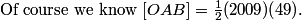DPatrick20:42:22
What is the area of OAP?
moplam20:42:49
1/2*2009*x
tinytim20:42:49
2009/2*(x_p)
Welovemath20:42:49
2009*x coordinate of p /2
DPatrick20:43:05
OAP has base OA = 2009 and height = (the x coordinate of P).
DPatrick20:43:17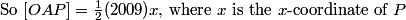DiscreetFourierTransform20:43:35
And similarly, [OQB] = 49/2 * (y-coordinate of Q)
DPatrick20:43:44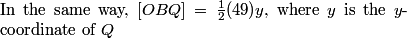DPatrick20:43:54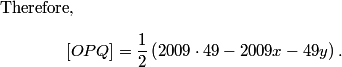DPatrick20:44:02
When is this an integer?
Mewto5555520:44:38
when exactly one of x or y is even
1=220:44:38
when x and y are of different parity
ytrewq20:44:38
when x and y are different parity
when x and y have opposite parity
DPatrick20:44:44
The quantity inside the parentheses must be even.
DPatrick20:44:48
So we need x and y to have different parities: one must be even and one must be odd.
DPatrick20:45:13
To get x and y of opposite parity, what do we need?
panjia12320:45:42
x even and y odd, or x odd or y even
moplam20:45:42
x_p and x_q to be both odd/even
sparkle12320:45:42
x coordinates of P and Q must have same parity
ytrewq20:45:42
x coordinate of P and Q to have the same parity
benzi45520:45:42
y has the opposite parity from Q's x-coordinate
DPatrick20:46:07
Each integer point on AB has coordinates of opposite parity. So we need to choose two points whose x-coordinates are the same parity, so the x of the first will be opposite parity than that of the y of the second.
moplam20:46:23
Thus we can choose from the 25 odd's or 25 even's
tinytim20:46:28
ytrewq20:46:28
2*25C2=2*300=600
DPatrick20:46:30
Right.
DPatrick20:46:39
We have C(25,2) choices of two points with both even x-coordinates.
DPatrick20:46:45
We have C(25,2) choices of two points with both odd x-coordinates.
DPatrick20:46:52
So 2*C(25,2) = 25*24 = 600 possible pairs of points.
DPatrick20:46:58
DPatrick20:47:42
I suspect a common mistake was forgetting that A and B themselves can be points.
DPatrick20:47:58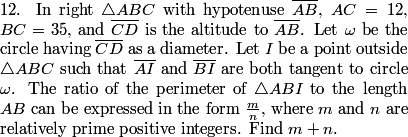DPatrick20:48:22
We start with a diagram. We label the points where AI and BI are tangent to the circle U and V, respectively.
DPatrick20:48:26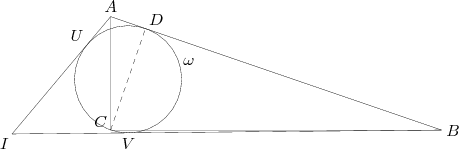mathmagus20:48:52
AB is 37, by pythagorean theorem
DPatrick20:48:57
We can find AB = 37 with the Pythagorean Theorem (or by knowing Pythagorean triples well).
DPatrick20:49:19
So, we're left with trying to find AI + BI. Can we find this easily?
bpgbcg20:49:47
Not really.
Kevin Zhang20:49:47
no...?
DPatrick20:50:08
Not directly, but we can find AU+BV easily.
Octahedr0n20:50:21
By Power of a Point, AU=AD, IU=IV, and BV=BD.
gh62520:50:21
DPatrick20:50:31
Because the diameter of the circle is perpendicular to AB, the circle is tangent to AB. We have AU = AD and BV = BD as tangents to the same circle from the same point. Therefore, AU + BV = AD +BD = AB.
DPatrick20:50:39
All that's left is to find UI (which equals VI).
DPatrick20:50:57
There's a slick way using some technical formulas...
skodali20:51:04
set heron's formula equal to semiperimeter times inradius
DPatrick20:51:17
This is the fastest if you know these formulas.
DPatrick20:51:32
If you know them, I probably don't need to work it out, and if you don't, I don't really want to explain them now.
DPatrick20:51:46
So I want to present a different solution that doesn't require this level of gadgetry.
DPatrick20:52:09
We can build a right triangle by drawing an altitude from the center of the circle, which we'll call O, to point U.
DPatrick20:52:14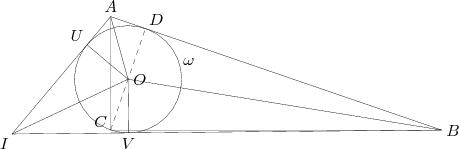shtsxc1220:52:37
Fermat160120:52:37
CD (diameter of cirlce) is 420/37
DPatrick20:53:13
We know that the area of the original triangle is [ABC] =(AC)(BC)/2 = (CD)(AB)/2, so CD = (AC)(BC)/AB = (12)(35)/37.
DPatrick20:53:28
We now have right triangle, IUO, and we have UO = CD/2. So, if we can find IO, or we can find one of the acute angles of the triangle (or a trig function of one of them), then we can finish.
tinytim20:53:59
tangent
DPatrick20:54:07
Yes: we can find tan <UOI, which we can use to find UI = UO tan <UOI.
DPatrick20:54:46
First, we note that AUO and ADO are congruent, as are OVB and ODB, and as are UOI and VOI.
DPatrick20:55:00
So those 6 central angles around O come in 3 congruent pairs.
DPatrick20:55:25
This means that <UOI = 180 - <AOD - <DOB.
DPatrick20:55:45
How does that help us find tan <UOI?
blackbelt1425320:56:13
tangent difference formula
DPatrick20:56:32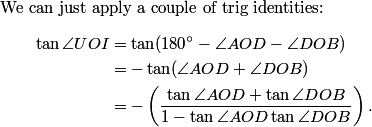DPatrick20:57:02
These are acute angles of right triangles. We have tan<AOD = AD/OD = AD/r, where r is the radius of the circle, and tan<BOD = BD/OD = BD/r.
DPatrick20:57:18
So we just plug them in and chug it out:
DPatrick20:57:27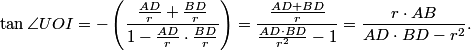DPatrick20:57:46
halelugia20:58:05
CD^2
blackbelt1425320:58:05
CD^2
brightzhu20:58:09
CD^2
benzi45520:58:11
4r^2?
DPatrick20:58:31
Right. AD*BD = (CD)^2 (by the similarity of ADC and CDB if you don't see it right away), so AD*BD = 4r^2.
DPatrick20:58:54
So now we have tan <UOI = AB/3r.
DPatrick20:59:11
Thus, what's UI?
BenM20:59:30
Ab/3
DPatrick20:59:41
Right, we have UI = UO tan <UOI = r tan<UOI = AB/3.
DPatrick20:59:58
And now we're essentially done: we just add up all the lengths in terms of AB>
DPatrick21:00:09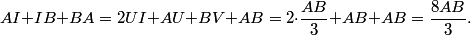blackbelt1425321:00:20
so perimeter is 8AB/3, and answer is 8+3=11
tinytim21:00:21
and we get 8/3=>011
DPatrick21:00:28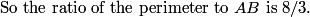DPatrick21:00:32
Thus the answer is 8+3 = 011.
moplam21:00:40
It sounds like it work for any right triangle
DPatrick21:01:00
Yes! If you followed carefully, we didn't actually need the numbers.
DPatrick21:01:19
Everything can be written in terms of AB.
DPatrick21:02:03
As I mentioned, there are more slick solutions using more complicated machinery (such as Heron's Formula); look it up and/or check the discussion forums if you're interested.
DPatrick21:02:14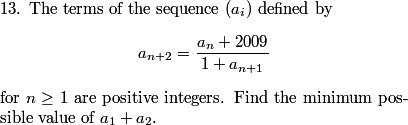DPatrick21:03:04
A possible strategy for this problem is to set the first two terms to be variables x and y, and then express all the subsequent terms in terms of x and y. However, the expressions become complicated very quickly, and do not shed much insight.
tinytim21:03:28
This problem was easy to guess correctly!
DPatrick21:03:45
Yeah, this problem is definitely guessable. You might not be able to prove your guess works though.
BenM21:03:49
if you made a hard to prove assumption
DPatrick21:03:51
Right.
DPatrick21:03:59
But let's not guess. Let's work it out. :)
DPatrick21:04:10
What's really glaringly ugly about the equation?
BenM21:04:22
2009
panjia12321:04:23
and the 2009
DPatrick21:04:34
That 2009 term is sitting there gumming up the whole works.
DPatrick21:04:47
So one plausible strategy is to try to eliminate it.
DPatrick21:04:56
Let's isolate the 2009 in the functional equation.
DPatrick21:05:06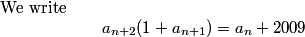DPatrick21:05:15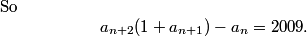DPatrick21:05:23
How does this help?
blackbelt1425321:05:34
invariant!
bpgbcg21:05:34
Invariant!
DPatrick21:05:57
Right, this gives us an invariant of the sequence, albeit a fairly ugly and hard-to-work with one right now.
DPatrick21:06:14
For any value of n, that expression is equal to 2009.
DPatrick21:06:16
How does that help?
FantasyLover21:06:50
try n=> n+1
DPatrick21:07:09
Sure. We can set two different values of this functional equation equal to each other. Specifically, we can replace n by n+1.
DPatrick21:07:29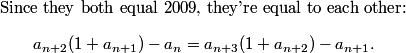DPatrick21:07:55
How does this help?
DPatrick21:08:36
This expression is hard to fathom as is, so we can start by expanding and putting everything to one side:
DPatrick21:08:42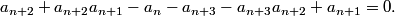DPatrick21:08:51
Can we refactor this in a nice way?
Yongyi78121:09:16
Factor terms with a_{n+2}?
DPatrick21:09:23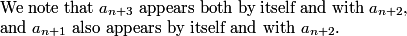DPatrick21:09:59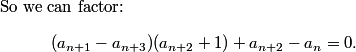DPatrick21:10:17
Let's rewrite this slightly:
DPatrick21:10:21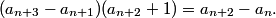DPatrick21:10:29
So what?
DiscreetFourierTransform21:11:00
We've got a difference of terms two apart in the first factor, and on the RHS
Yongyi78121:11:00
The RHS is the first factor of the LHS with n => n+1
DPatrick21:11:06
We see the same sort of expression on both sides.
DPatrick21:11:13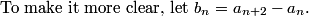DPatrick21:11:27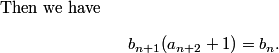DPatrick21:11:50
What does that tell us?
bpgbcg21:12:06
b_n is always an integer.
DPatrick21:12:23
How does that help draw some sort of conclusion?
projectstartrek21:12:43
b_n+1 divides b_n
benzi45521:12:47
so therefore each term of b is a factor of all previous terms?
DPatrick21:13:36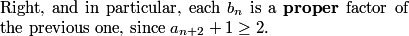DPatrick21:14:02
But we can't have an infinite sequence of proper factors, unless...
Yongyi78121:14:12
The only way this is possible is if b_n = 0 for all n
DPatrick21:14:18
Right.
DPatrick21:14:41
If b_1 is nonzero, then b_2 has to be a proper factor of b_1, and then b_3 has to be a proper factor of b_2, and so on.
DPatrick21:14:51
But we only have finitely many factors, so this can't go on forever.
DPatrick21:15:01
The only possiblity is that all the b's are 0.
Kevin Zhang21:15:12
So a_n+2 = a_n
DiscreetFourierTransform21:15:12
a_n+2 = a_n
tinytim21:15:12
so a_(n+2)=a_n for all n
DPatrick21:15:16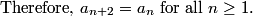DPatrick21:15:21
(This type of argument is sometimes called infinite descent.)
DPatrick21:15:34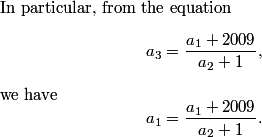tinytim21:16:05
and a_1a_2=2009
blackbelt1425321:16:05
so a_1a_2=2009
Yongyi78121:16:05
Multiply both sides by (a_2+1) to get a_1*a_2=2009
DPatrick21:16:11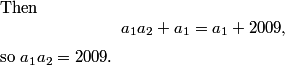DPatrick21:16:18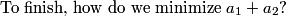Bobby Jacobs21:16:38
41, 49 so it is 090
moplam21:16:38
41+49
ytrewq21:16:38
41,49
panjia12321:16:38
they have to be as close to each other as possible
DPatrick21:16:44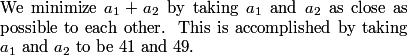DPatrick21:16:54
Thus the answer is 41+49 = 090.
DPatrick21:17:40
The reason many people thought it was "guessable" is that since that 2009 is stuck there, you might trying guessing that a_1 and a_2 are factors of 2009.
DPatrick21:18:03
Computing then quickly shows that a_3 = a_1 in this case, and the rest follows.
DPatrick21:18:14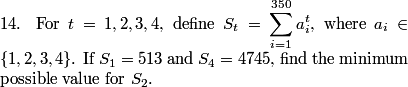DPatrick21:18:49
What is the key data that we need to worry about?
grn_trtle21:19:16
the number of 1s, 2s, 3s and 4s
ytrewq21:19:17
then number of times each value 1,2,3,4 appears
DPatrick21:19:23
We don't care what order the a_i are in, we only care about how many of each number there are.
DPatrick21:19:31
So let: w be the number of 1s among the a's x be the number of 2s among the a's y be the number of 3s among the a's z be the number of 4s among the a's
DPatrick21:19:44
What equations can we write?
miller4math21:19:57
w+x+y+z=350
ytrewq21:20:01
w+x+y+z=350
mathtyro21:20:01
w+x+y+z = 350
DPatrick21:20:08
There are 350 a's, so we must have w+x+y+z = 350.
brightzhu21:20:20
w+2x+3y+4z=513
miller4math21:20:21
w+2w+3y+4z=513
bpgbcg21:20:21
w+2x+3y+4y=513
DPatrick21:20:27
Computing S_1 gives w + 2x + 3y + 4z = 513.
projectstartrek21:20:40
w+2^4x+3^4y+4^4z=4745
FantasyLover21:20:40
w+16x+81y+256z=4745
miller4math21:20:40
w+16x+81y+256z=4745
DPatrick21:20:47
Computing S_4 gives w + 16x + 81y + 256z = 4745.
DPatrick21:20:56
And what is the value we're trying to minimize?
blackbelt1425321:21:18
w+4x+9y+16z
mathtyro21:21:18
w+4x+9y+16z
DPatrick21:21:28
S_2, which is equal to w + 4x + 9y + 16z
DPatrick21:21:35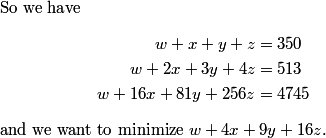DPatrick21:22:09
There are a number of ways to plow through this algebra... I will try to guide us through what I think is the simplest.
Yongyi78121:22:24
Subtract (1) from (2) and (3) to get 2 equations in 3 variables
DPatrick21:22:52
I'd go even one step further. I'd subtitute for w using the first equation w = 350 - x - y - z...
DPatrick21:23:00
...which is the essentially the same as subtracting it...
DPatrick21:23:08
...but it all the expression, including the S_2 expression.
DPatrick21:23:20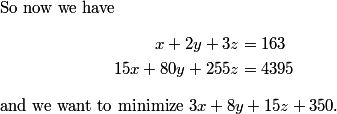DPatrick21:23:42
Keeping track of what we want to minimize is going to be useful for the last step.
Kongster21:23:52
now do the same with the x
projectstartrek21:23:52
do the same thing with x again
DPatrick21:24:00
Right: now we can use x from the top expression to sub into the others. x = 163 - 2y - 3z.
DPatrick21:24:11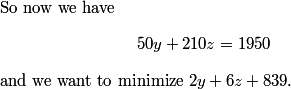DPatrick21:24:36
There's nothing left to "solve", so what now?
miller4math21:24:41
divide (1) by 10
DPatrick21:24:47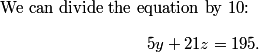DPatrick21:24:59
What are the possiblilites?
DPatrick21:25:13
(with one l, preferably.)
DiscreetFourierTransform21:25:18
remember that y and z have to be integers
DPatrick21:25:24
nonnegative integers in fact.
DPatrick21:25:36
That 21 sticks out.
DPatrick21:25:58
It's the only term that's not a multiple of 5 automatically.
tinytim21:26:02
z must be 5
mathmagus21:26:02
z has to be 5
DPatrick21:26:07
I wouldn't say that yet...
mathtyro21:26:20
z could also be 0
Welovemath21:26:21
it could be zero
DPatrick21:26:34
Right. z must be a multiple of 5, because the other two terms are. z >= 10 is too big. So z must be 0 or 5.
DPatrick21:26:50
We could try them both and see which gives a bigger S_2, or...
DPatrick21:27:19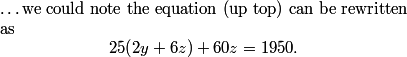DPatrick21:27:37
So 2y+6z is minimized when 60z is maximized, hence we want the largest possible value of z.
blackbelt1425321:27:51
(z,y)=(5,18)
DPatrick21:28:09
Right; to finish, z=5 gives y=18, and we can plug these in to finish.
DPatrick21:28:24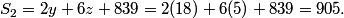DPatrick21:28:39
DPatrick21:28:46
(With sufficient time, I would go back and solve for w and x and double-check that it all works.)
DiscreetFourierTransform21:29:04
Last problem!
DPatrick21:29:17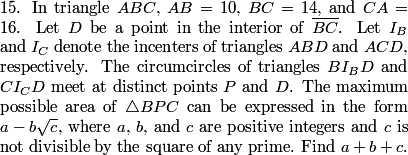BenM21:29:44
draw it
ytrewq21:29:44
draw a diagram
DPatrick21:29:52
DPatrick21:29:55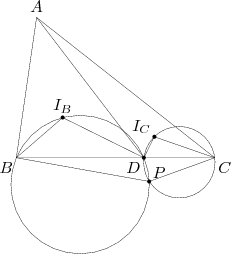DPatrick21:30:04
(I left out the incircles to keep the diagram from being a complete nightmare.)
DPatrick21:30:20
As a first step, let's make sure we understand what we're going after. We want to maximize the area of triangle BPC.
DPatrick21:30:45
In a very vague sense, where do we want P to maximize [BPC]?
BenM21:30:57
which means maximize distance of p from BC
blackbelt1425321:30:57
P is farthest away from BC
DiscreetFourierTransform21:30:57
Far from BC
bpgbcg21:30:57
As far from BC as possible.
DPatrick21:31:06
Right: since BC is fixed, the area of BPC is maximized when P is as far from BC as possible.
DPatrick21:31:24
So, we now have a target: get as much information about P as possible.
DPatrick21:31:40
Before we start scribbling down all sorts of information, let's focus a bit. We have a varying set-up, so what should we be looking for?
DPatrick21:32:16
In other words, this diagram has a lot of moving parts, since we can slide D back and forth along BC.
DiscreetFourierTransform21:32:26
Angles that are fixed.
DPatrick21:32:54
Right: a good thing to look for is angles (or sums of angles) that don't change as we slide D around.
DPatrick21:33:09
(Sliding D around appears to change all the lengths in the problems except for the sides of ABC, so that's not to promising to look for lengths that are fixed.)
DPatrick21:33:19
Moreover, the problem involves angle bisectors (because of the incenters), and usually that means looking at angles rather than lengths.
DPatrick21:33:47
Are there any "moving" angles whose measures are fixed as we slide D back and forth?
bpgbcg21:34:03
DBI_B and DCI_C are fixed.
Farnak21:34:13
I_B,D and I_C,D are angle bisectors so angle I_B,D,I_c is right
DPatrick21:34:29
That's a good place to start; let me rewrite it in LaTeX so it's readable :)
DPatrick21:34:45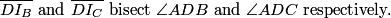DPatrick21:35:04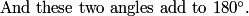DPatrick21:35:10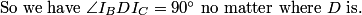DPatrick21:35:29
But sadly, it's not clear how that's helpful, so we keep hunting.
jli21:35:41
BPC
DPatrick21:35:47
We'd like to learn more about P, so we should investigate angle BPC. Any suggestions how to do so?
jli21:36:07
happysunnyshine21:36:07
connect DP
DPatrick21:36:23
We can break <BPC into <BPD+<DPC, which we do to enable us to use the circumcircles in the problem. So we add DP to our diagram:
DPatrick21:36:28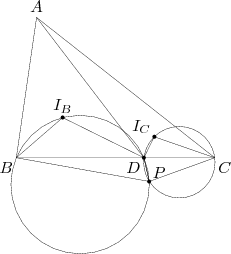DPatrick21:36:55
Now we have a couple of cyclic quadrilaterals, so that should give us some info about the various angles.
happysunnyshine21:37:49
bpc=360-(I_b+I_c)
DPatrick21:38:04
I think so...let's see.
DPatrick21:38:27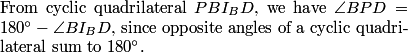DPatrick21:38:42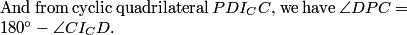DPatrick21:39:32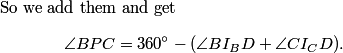DPatrick21:39:46
What do we know about those two angles inside the parentheses?
BenM21:40:38
they are related to BAC
DPatrick21:41:14
Right, we can use the incircles to break them down in terms of the angles of BAC.
happysunnyshine21:41:25
because I_b and I_c are incentres
DPatrick21:41:34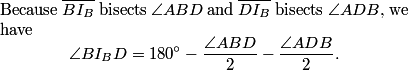DPatrick21:42:09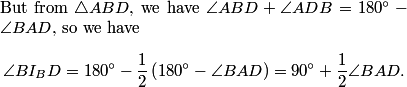DPatrick21:42:35
(If you're not used to using incenters to do angle-chasing like this, you may want to go back later and work out these calculations on your own.)
DPatrick21:42:54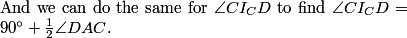DPatrick21:43:30
So if we take these last two statements, and plug them into the equation for BPC that I just popped up to the top, what can we conclude?
BenM21:44:05
BPC is constant
ytrewq21:44:05
BPC=180-1/2BAC
DPatrick21:44:21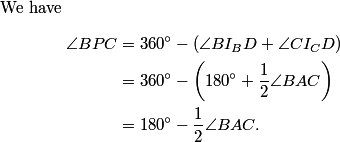DPatrick21:44:40
Aha! <BPC is fixed, regardless of where D is located on the segment!
BenM21:44:48
so the locus it traces out is an arc
DPatrick21:44:57
Happy day! This tells us that P is on a fixed arc from B to C.
DPatrick21:45:02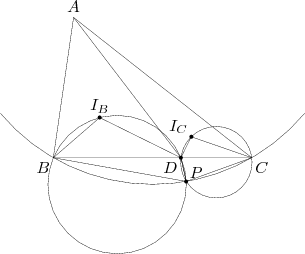BenM21:45:27
so the low point must be in the middle
bpgbcg21:45:27
so the distance from P to BC is maximized when P is on the perpendicular bisector of BC.
DPatrick21:45:35
To maximize the distance from P to BC, P must be at the midpoint of this arc. (To see that the midpoint is attainable, note that as D varies from B to C along BC, P moves continuously from B to C along the arc.)
DPatrick21:45:44
DPatrick21:45:55
What would be useful to know next?
moplam21:46:47
m andgle BAC
DPatrick21:47:21
Since we probably need the actual value of angle BPC to compute the area we want, it'd be nice to know angle BPC's values, and we can get it if we know angle BAC.
moplam21:47:26
law of consine can find angle BAC
Welovemath21:47:27
laws of cosines
DPatrick21:47:42
We can apply the Law of Cosines to ABC since we were given all the lengths.
blackbelt1425321:47:51
it is 60 degrees by law of cosines
moplam21:47:51
it's 60 degrees=.=
DPatrick21:48:02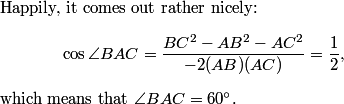DPatrick21:48:27
Therefore, <BPC = 180 - (1/2)(60) = 150 degrees.
benzi45521:48:59
one step left
DPatrick21:49:11
Right. To finish, we want the area of an isosceles triangle with base 14 and angle 150 degrees.
blackbelt1425321:49:25
well now we just have to bash tan 75 to get the answer
DPatrick21:49:46
That's one way, or we can use Law of Cosines again on triangle BPC to compute BP=CP.
DPatrick21:49:58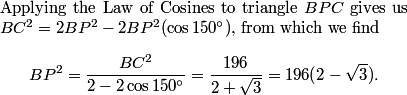DPatrick21:50:36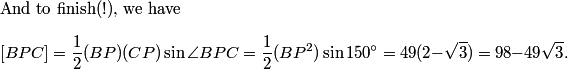DPatrick21:51:00
(I should say it is slightly quicker to use tan(75) if you know it.)
BenM21:51:06
Welovemath21:51:06
sum is 150
DPatrick21:51:08
So the answer is 98+49+3 = 150.
DPatrick21:51:16
A worthy #15 I think.
DPatrick21:51:31
That's it for the Math Jam. Just a shade under 3 hours. :)
DPatrick21:51:58
Note that the AIME II is on April 1, and we'll be back for the AIME II Math Jam on April 3.
DPatrick21:52:11
Thanks for coming and participating...have a good night! :)
benzi45521:52:41
what would you say of the difficulty of the exam as a whole?
DPatrick21:52:59
If you're solid in counting and geometry, it was probably (relatively) easier than usual.
DPatrick21:53:09
If you don't like counting or geometry, then probably hard.
geoffreywong21:53:31
any guess on the index score?
DPatrick21:53:38
I make it a policy not to speculate.
DPatrick21:53:49
There's really no point in trying to predict it.
gihun957821:56:08
Do you know any bookstore where I can get Art of Problem Solving Textbooks (in the North Hollywood, Los Angeles, CA)?
DPatrick21:56:32
Not in North Hollywood, but there's a bookstore on Wilshire Blvd that has our stuff.
Kongster21:56:36
when is the usamo
DPatrick21:56:43
April 28 and 29.
all4math21:57:30
What is the Law of Cosines???:-o
kevinw21:58:06
would someone who took the AMC10 and scored a 6 on this have a shot at the USAMO?
DPatrick21:58:15
Again, I make it a policy not to speculate.
DPatrick21:58:28
Past years' stats are on the AMC website.
tinytim21:58:32
If the AIME I has an emphasis on a certain subject, is it likely that the AIME II will have an emphasis on the same subject?
DPatrick21:58:35
I doubt it.
benzi45521:58:46
when is the IMO?
DPatrick21:59:00
I don't know but I suspect that is easily obtainable information via a very simple web search.
benzi45521:59:29
This is the wrong place to ask, but how good are the scanning machines they use? I made some erasures and I hope the machine picks up the right bubbles.
DPatrick21:59:32
I haven't a clue.
DPatrick21:59:50
The AMC Teacher's Manual has provisions for getting your paper hand-rescored (for a fee); check their website.
DPatrick21:59:58
FYI, the AMC website is www.unl.edu/amc
BenM22:00:11
how should you transition from practicing AIME to olympiad problems if every single USAMO question you look at seems impossible?
DPatrick22:00:20
DPatrick22:00:52
Canada and the UK also have easier Olympaid-style contests.
orl22:00:57
IMO will be in Germany from 10th to 22nd June. http://www.imo2009.de/imo/index.php?option=com_content&view=article&id=12&Itemid=20&lang=en
DPatrick22:00:59
Thanks.
DPatrick22:01:36
OK, I've been for over 3 hours, time for me to eat dinner.
DPatrick22:01:37
Bye!Sign In